﻿ Solution of Partial Integro-Differential Equations Involving Mixed Partial Derivatives by Laplace Substitution MethodPublications are Open
Access in this journal
Article Versions
Export Article
• Normal Style
• MLA Style
• APA Style
• Chicago Style
Research Article
Open Access Peer-reviewed

### Solution of Partial Integro-Differential Equations Involving Mixed Partial Derivatives by Laplace Substitution Method

S.S. Handibag , B. D. Karande, S. V. Badgire
American Journal of Applied Mathematics and Statistics. 2018, 6(6), 272-280. DOI: 10.12691/ajams-6-6-9
Received October 01, 2018; Revised December 03, 2018; Accepted December 19, 2018

### Abstract

This paper studies the Laplace substitution method for nonlinear partial integro-differential equations involving mixed partial derivatives and further applications of same method for coupled nonlinear partial integro-differential equations involving mixed partial derivatives.

### 1. Introduction

The study of integro-differential equations started in the fifties with the works of Getoor, Blumenthal, and Kac, among others. Due to the relation with stochastic processes, they studied Dirichlet problems of the form(1.1)

being L the infinitesimal generator of some stochastic process in the simplest case, L would be the fractional Laplacian. In 1959, the continuity up to the boundary of solutions was established, and also some spectral properties of such operators 1. For the fractional Laplacian the asymptotic distribution of eigenvalues was obtained, as well as some comparison results between the Green's function in a domain and the fundamental solution in the entire space 2. Later, sharp decay estimates for the heat kernel of the fractional Laplacian in the whole Rn were proved 3, and an explicit formula for the solution of(1.2)

was found 4, 5. Moreover, Green's function and the Poisson kernel for the fractional Laplacian in the unit ball B1 were also explicitly computed by Getoor 6 and Riesz 7, respectively.

Integro-differential equations arise naturally in the study of stochastic processes with jumps, and more precisely of Levy processes. This type of processes, well studied in Probability, is of particular interest in Finance, Physics, or Ecology. Moreover, integro-differential equations appear naturally also in other contexts such as Image processing, Fluid Mechanics, and Geometry.

To a great extent, the study of integro-differential equations is motivated by real world applications. Indeed, there are many situations in which a nonlocal equation gives a significantly better model than a PDE, as explained next.

Integro-differential equations appear also in Ecology. Indeed, optimal search theory predicts that predators should adopt search strategies based on long jumps where prey is sparse and distributed unpredictably, Brownian motion being more efficient only for locating abundant prey; see 8, 9, 10.

The partial integro-differential equation is a part of integro-differential equations. The number of mathematicians has been work successfully on the methods for solving partial integro-differential equations. But yet the date no one work on partial integro-differential equations involving mixed partial derivatives.

In this paper we have concentrated on the nonlinear partial integro-differential equations involving mixed partial derivatives and develop a solution method for nonlinear partial integro-differential equations, namely LSM. In section 2, we have given the description of LSM for nonlinear partial integro-differential equation and in section 3; we have solved two nonlinear partial integro-differential equations by using LSM finally in the last section 4 we have given the conclusion of this paper.

### 2. Method of Solution

In this section, we have given the description of Laplace substitution method 11, 12, 13 for nonlinear partial Integro-differential equations involving mixed partial derivatives. In the same section, we have consider the following general form of NPIDE,(2.1)

with initial conditions,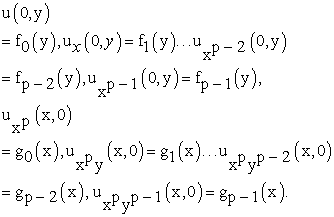(2.2)

Where K(x, y) and f(x, y) are given functions, α is a constant,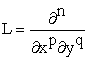(p and q are positive integers with p + q = n), Ru(x, y) and R*u(x, y) are general linear terms, Nu (x, y) and N*u (x, y) are nonlinear terms. Equation (2.1), we can write in the following form,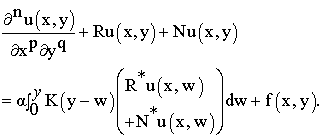In above nonlinear partial integro-differential equation we have considered x as an independent variable under integral sign. Therefore here we are going to use the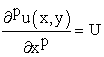substitution in equation (2.1), we getTaking Laplace transform of above equation with respect to y, we get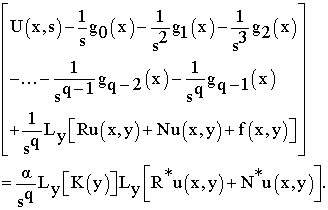Taking inverse Laplace transform of above equation with respect to y, we get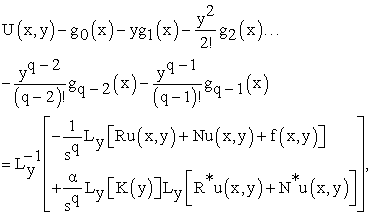Re-substitute the value of U(x, y) in above equation, we getTaking Laplace transform of above equation with respect to x, we get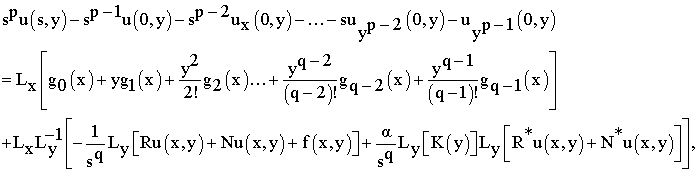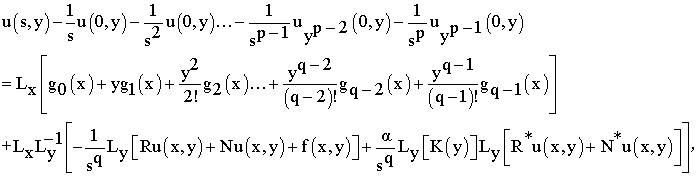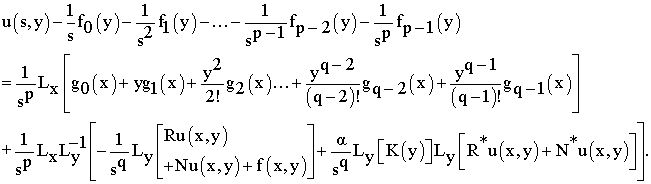Taking inverse Laplace transform of above equation with respect to x, we get(2.5)

Suppose that solution of equation (7.2.1) is in series form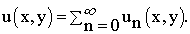(2.6)

The nonlinear terms, which are appeared in equation (2.1), we can decompose them by using Adomian polynomial(2.7)

In above equation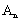andare Adomian polynomials depending on the components u0 (x, y), u1 (x, y)………, un (x, y), n ≥ 0 of series solution (2.6). Substitute equations (2.6) and (2.7), we getComparing both sides of above equations, we get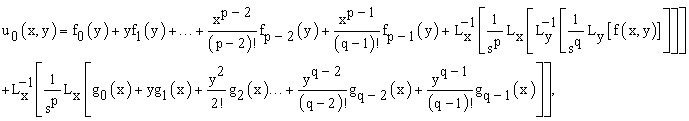From the above equations, we get the following recursive relation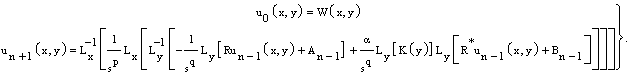(2.9)

WhereFrom the above recursive relation, we can find the components of the series solution (2.6). In the recursive relation (2.9), some time we will use the technique of Modified Laplace Substitution method, which was we have explained in 11, 12, 13.

### 3. Application of Laplace Substitution Method

In this section, we have given the applications of Laplace substitution method for nonlinear partial integro-differential equations. In the same section we have solved two problems by LSM. In both problems for our convenience, we have used Modified Laplace substitution method.

Example 3.1: Consider the following nonlinear partial Integro-differential equation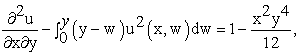(3.1)

with initial conditions,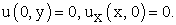(3.2)

As we have explained in section (2), y is an independent variable under integral sign. Therefore we have used substitution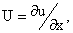in above equation. Then the above equation becomes,Taking the Laplace transformation of above equation with respect to y, we get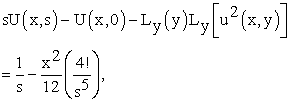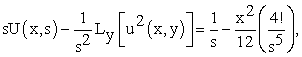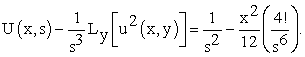Taking inverse Laplace transform of above equation with respect to y, we getRe-substitute the value U(x, y) in above equation, we getTaking Laplace transform of above equation with respect to x, we get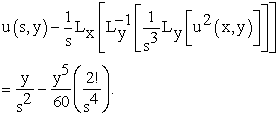Taking inverse Laplace transform of above equation with respect to x, we get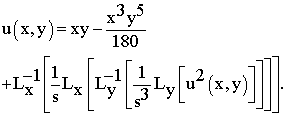(3.3)

Suppose that,(3.4)

is a series solution of equation (3.1). The nonlinear term appeared in equation (3.1); we can decompose it by using Adomian polynomial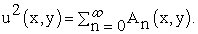(3.5)

Where An is an Adomian polynomial depending on the components u0(x, y), u1(x, y), u2(x, y)……un(x, y), n ≥ 0. In this thesis we have found the Adomian polynomials of equation (3.5) in last some problems. Substitute equations (3.4) and (3.5) in equation (3.3), we get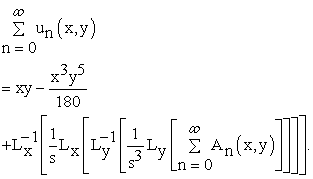Comparing both sides of above equation, during the comparison we have used MLSM, by splitting the source term in two part, we get the following equations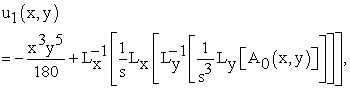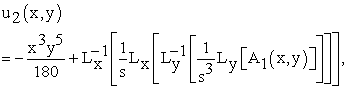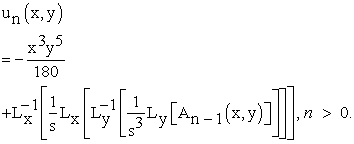From the above equations, we get the following recursive relation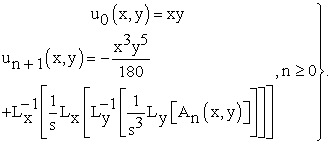(3.6)

The components of series (3.4), with the help of above recursive relation are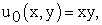This implies that,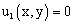. From the recursive relation we observe that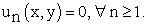Therefore the exact solution of equation (7.3.1) after substitution of all values of components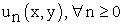in equation (7.3.4) is,We have verified this through the substitution.

Example 3.2: Consider the following nonlinear partial Integro-differential equation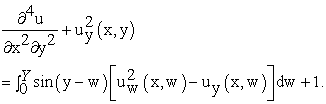(3.7)

With initial conditions,(3.8)

Let we use the substitutionin above equation, we get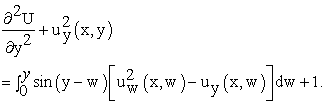Taking Laplace transform of above equation with respect to y, we get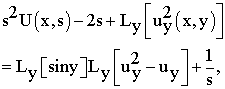Taking inverse Laplace transform of above equation with respect to y, we get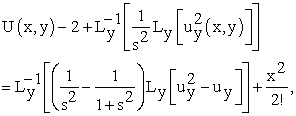Re-substitute the value of U(x, y) in above equation, we get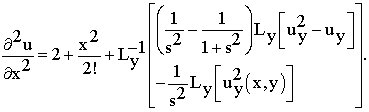Taking Laplace transform of above equation with respect to x, we get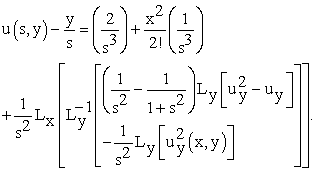Taking inverse Laplace transform of above equation with respect to x, we get(3.9)

Suppose that,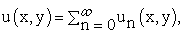(3.10)

is a required solution of equation (3.7). The nonlinear term appeared in equation (3.7), we decompose it by using Adomian polynomial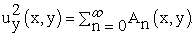(3.11)

Where An is an Adomian polynomial of components un(x, y), n ≥ 0 of series (3.10). Substitute equations (3.10) and (3.11) in equation (3.9), we getComparing both sides of above equations, we get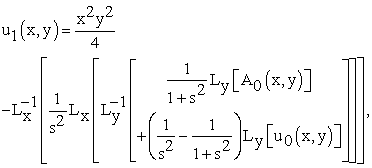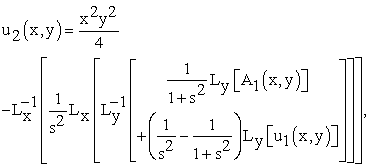From the above equations, we get the following recursive relation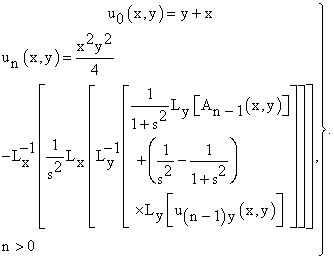(3.12)

Let we find the components of series (3.10) by using above recursive relation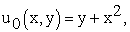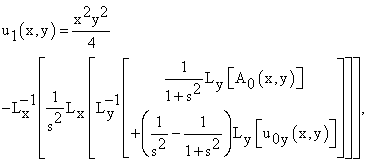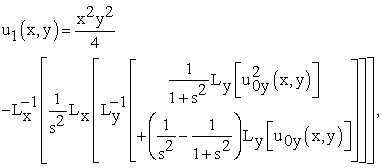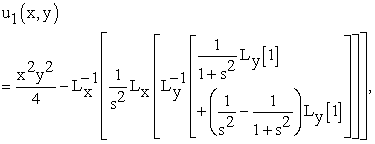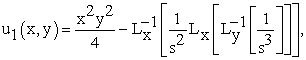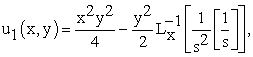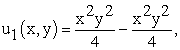From the recursive relation (3.12), and value of u1(x, y) = 0, we get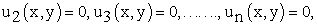Substitute all values ofin equation (3.10), we get the required solution of equation (3.7),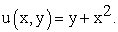This is an exact solution of equation (3.7) with initial conditions (3.8). We have verified this through the substitution.

### 4. Conclusion

This paper is nothing but the one more achievement of LSM. In 11, 12, 13 we have describe LSM for different order linear and nonlinear partial differential equations involving mixed partial derivatives and applied it successfully to number of linear and nonlinear problems. In this paper we have applied same method for nonlinear partial integro-differential equations involving mixed partial derivatives. It gives the exact solution with less computation. The main advantage of this method is the fact that it gives the analytical solution. In the above examples we observed that the LSM with the initial conditions yield an exact only in a few iterations. It is also worth noting that the advantage of the decomposition methodology displays a fast convergence of the solutions. The illustrations shows the dependence of the rapid convergence depend on the character and behavior of the solutions just as in a closed form solutions. It is shown that this method is a promising tool for the linear and nonlinear partial integro-differential equations involving mixed partial derivatives.

### References

  R. K. Getoor, Markov operators and their associated semi-groups, Pacific J. Math. 9 (1959), 449-472. In article View Article  R. M. Blumenthal, R. K. Getoor, The asymptotic distribution of the eigenvalues for a class of Markov operators, Pacific J. Math. 9 (1959), 399-408. In article View Article  R. M. Blumenthal, R. K. Getoor, Some theorems on stable processes, Trans. Amer. Math. Soc. 95 (1960), 263-273. In article View Article  R. K. Getoor, First passage times for symmetric stable processes in space, Trans. Amer. Math. Soc.101 (1961), 75-90. In article View Article  M. Kac, H. Pollard, Partial sums of independent random variables, Canad. J. Math 11 (1950), 375-384. In article View Article  R. M. Blumenthal, R. K. Getoor, D. B. Ray, On the distribution of first hits for the symmetric stable processes, Trans. Amer. Math. Soc. 99 (1961), 540-554. In article  M. Riesz, Integrales de Riemann-Liouville et potentiels, Acta Sci. Math. Szeged, 1938. In article  N. E. Humphries et al., Environmental context explains Levy and Brownian movement patterns of marine predators, Nature, 465 (2010), 1066-1069. In article View Article  PubMed  A. M. Reynolds, C. J. Rhodes, the Levy fight paradigm: Random search patterns and mechanisms, Ecology 90 (2009), 877-887. In article View Article  PubMed  G. M. Viswanathan et al., Levy fight search patterns of wandering albatrosses, Nature 381 (1996), 413-415. In article View Article  S. S. Handibag, B.D. Karande, An Application for nonlinear Partial Differential Equations Involving Mixed Partial Derivatives by LSM, AIP Conference Proceeding 1637, 384, 2014. In article  S. S. Handibag, B.D. Karande, An Extended Application of Laplace Substitution Method, International Journal of scientific and Innovative Mathematical Research, Volume 3, Special Issue 2015, 568-574, ISSN: 2347-307X, (Print), www.arcjournals.org. In article View Article  S. S. Handibag, B. D. Karande, Laplace Substitution Method for nth order Linear and Nonlinear Partial Differential Equations Involving Mixed Partial Derivatives, International Research Journal of Engineering and Technology, Volume 2, Issue 9, 2015, P-ISSN: 2395-0072, e-Issn: 2395-0056. In articleThis work is licensed under a Creative Commons Attribution 4.0 International License. To view a copy of this license, visit http://creativecommons.org/licenses/by/4.0/